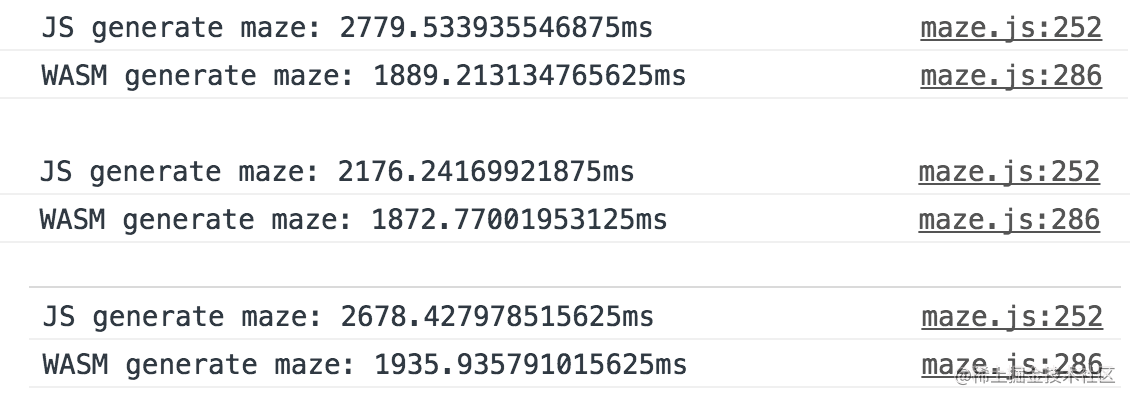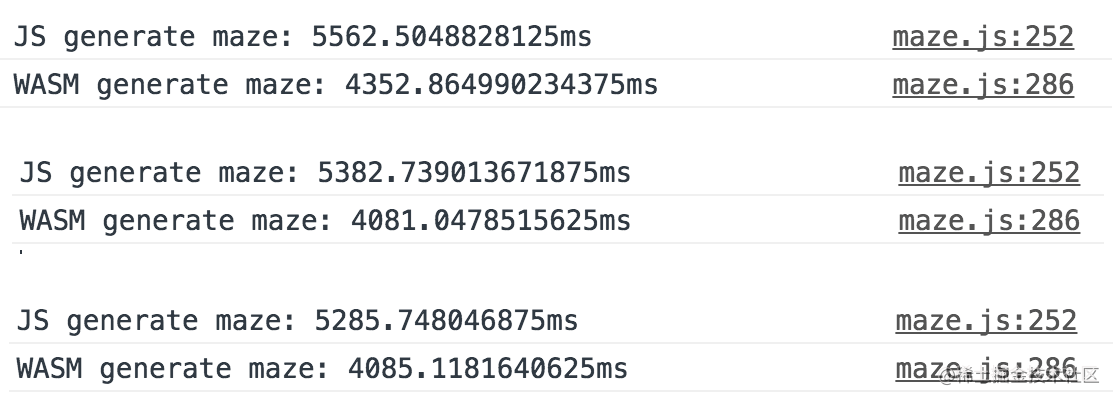# 用 Canvas + WASM 画一个迷宫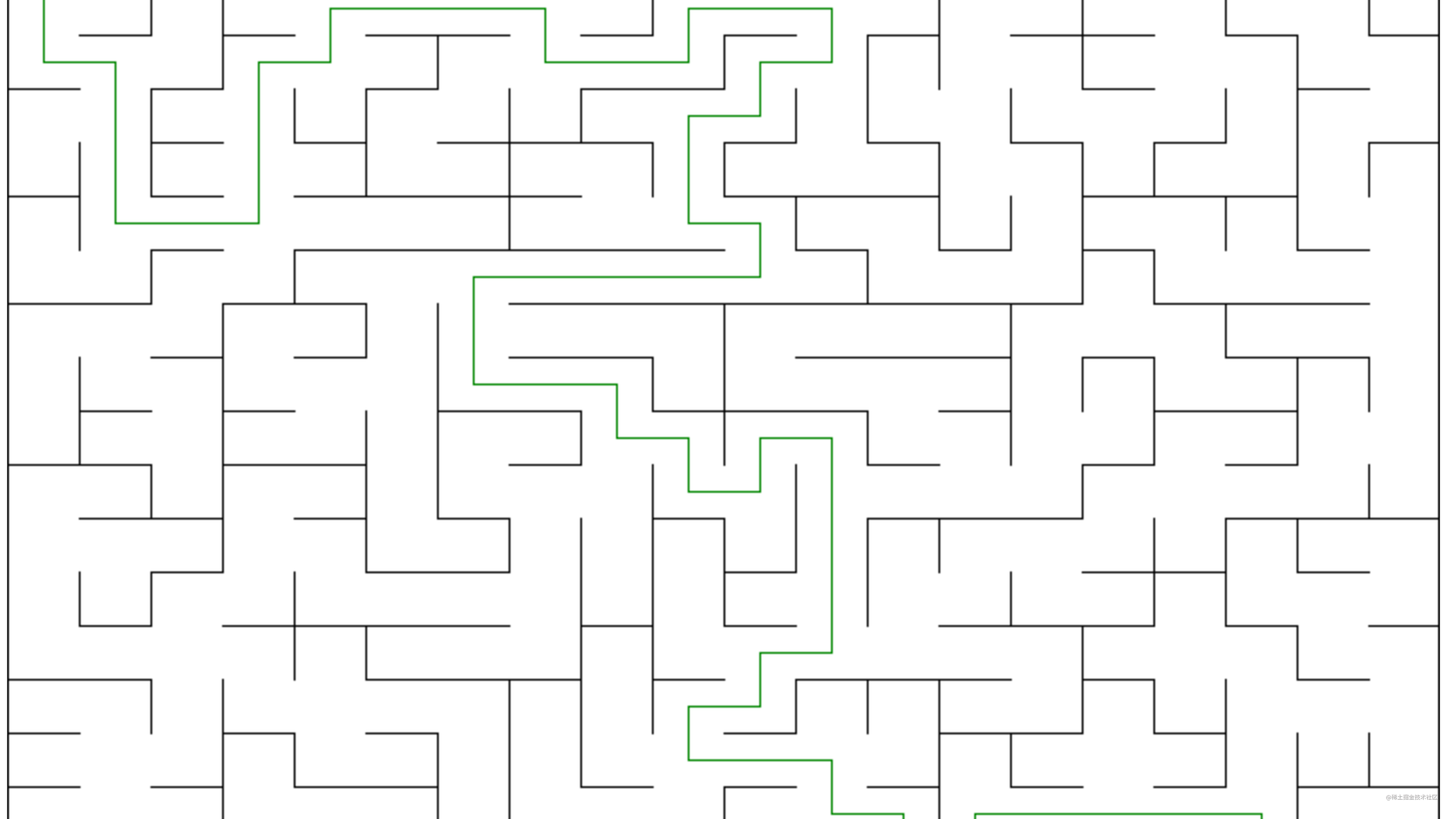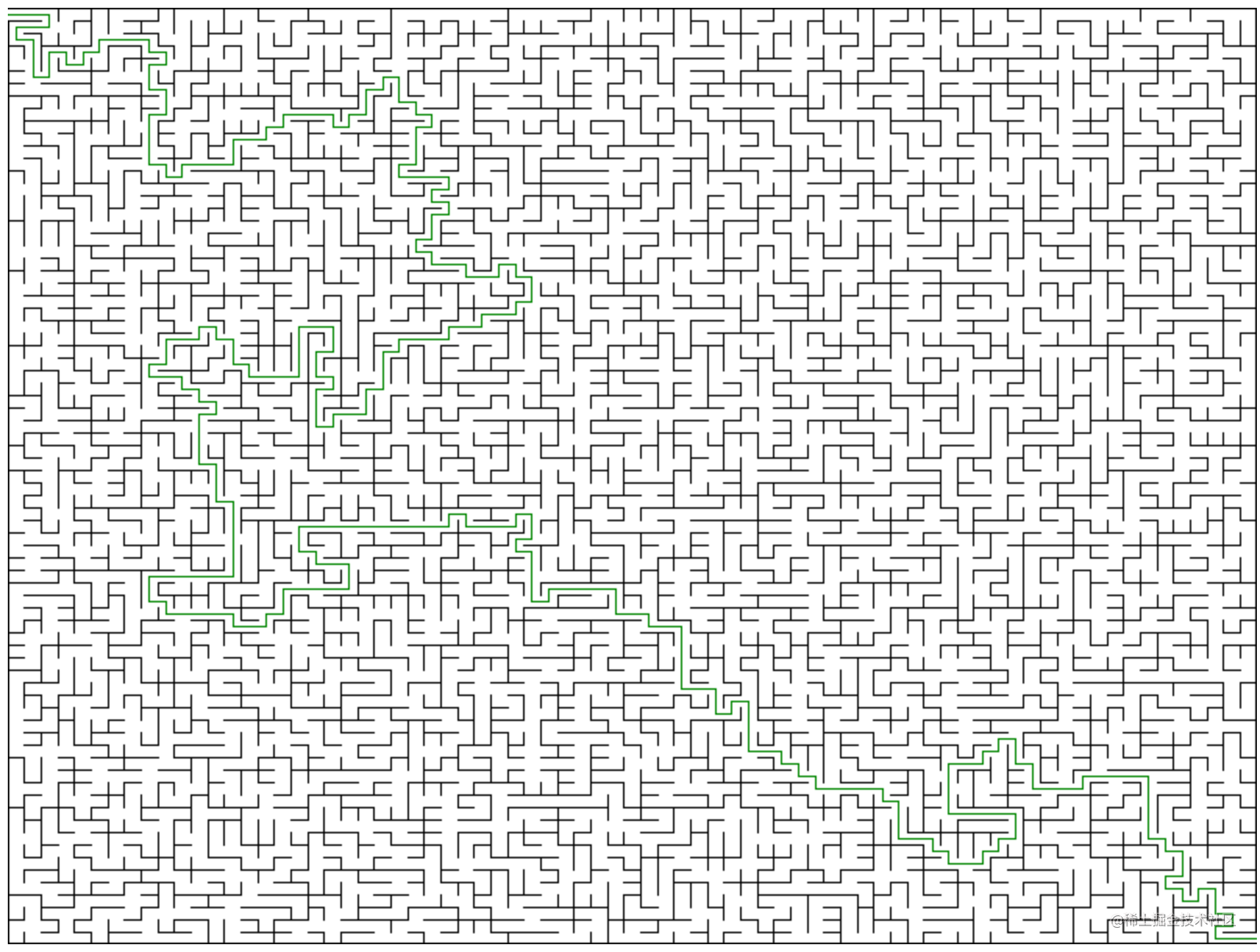## 1. 用连通集算法生成迷宫

（1）生成100个格子，每个格子都不相通

（2）随机选取相邻的两个格子，可以是左右相邻或者是上下相邻，判断这两个格子是不是处于同一个连通集，即能否从其中一个格子走到另外一个格子，如果不能，就拆掉它们中间的墙，让它们相连，处于同一个连通集。

（3）重复第二步，直到第一个格子和最后一个格子相连。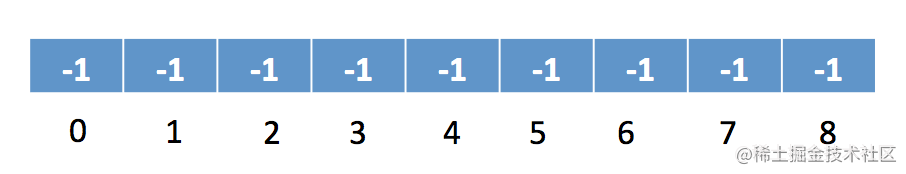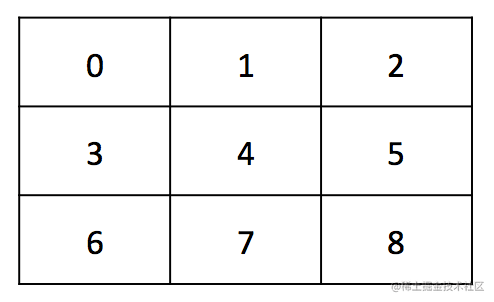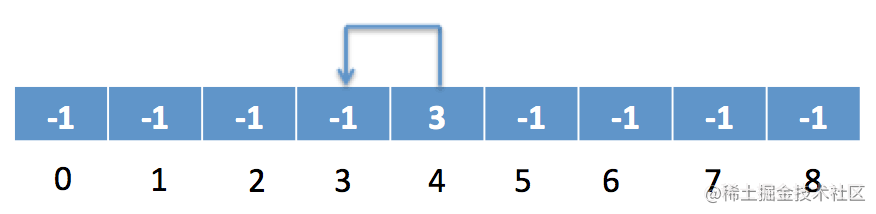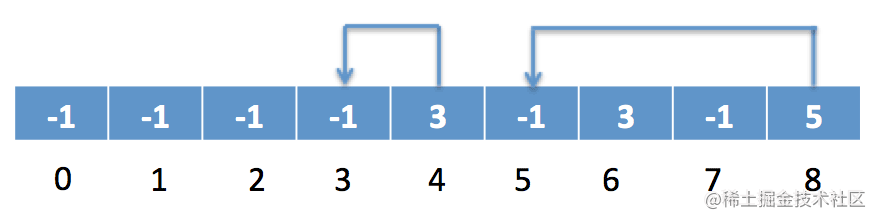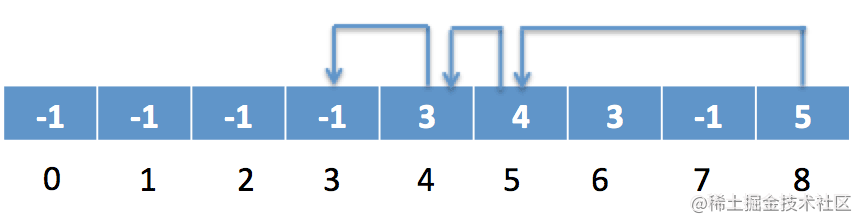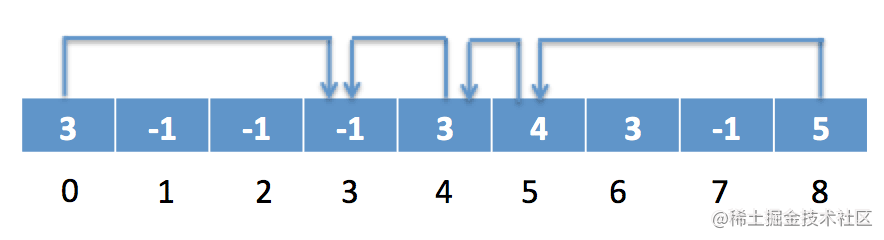``````class UnionSet{
constructor(size){
this.set = new Array(size);
for(var i = this.set.length - 1; i >= 0; i--){
this.set[i] = -1;
}
}
union(root1, root2){
this.set[root1] = root2;
}
findSet(x){
while(this.set[x] >= 0){
x = this.set[x];
}
return x;
}
sameSet(x, y){
return this.findSet(x) === this.findSet(y);
}
unionElement(x, y){
this.union(this.findSet(x), this.findSet(y));
}
}复制代码``````

``````class Maze{
constructor(columns, rows, cavans){
this.columns = columns;
this.rows = rows;
this.cells = columns * rows;
//存放是连通的格子，{1: [2, 11]}表示第1个格子和第2、11个格子是相通的
this.unionSets = new UnionSet(this.cells);
this.canvas = canvas;
}
}复制代码``````

Maze类再添加一个生成迷宫的函数，如下代码所示：

``````//生成迷宫
generate(){
//每次任意取两个相邻的格子，如果它们不在同一个连通集，
//则拆掉中间的墙，让它们连在一起成为一个连通集
var cellPairs = this.pickRandomCellPairs();
if(!this.unionSets.sameSet(cellPairs, cellPairs)){
this.unionSets.unionElement(cellPairs, cellPairs);
}
}
}复制代码``````

``````//取出随机的两个挨着的格子
pickRandomCellPairs(){
var cell = (Math.random() * this.cells) >> 0;
//再取一个相邻格子，0 = 上，1 = 右，2 = 下，3 = 左
var neiborCells = [];
var row = (cell / this.columns) >> 0,
column = cell % this.rows;
//不是第一排的有上方的相邻元素
if(row !== 0){
neiborCells.push(cell - this.columns);
}
//不是最后一排的有下面的相邻元素
if(row !== this.rows - 1){
neiborCells.push(cell + this.columns);
}
if(column !== 0){
neiborCells.push(cell - 1);
}
if(column !== this.columns - 1){
neiborCells.push(cell + 1);
}
var index = (Math.random() * neiborCells.length) >> 0;
return [cell, neiborCells[index]];
}复制代码``````

``````addLinkedMap(x, y){
}
}
}复制代码``````

``````findSet(x){
if(this.set[x] < 0) return x;
return this.set[x] = this.findSet(this.set[x]);
}复制代码``````

union函数也可以做一个优化，findSet可以把树的高度改小，但是在没有改小前的union操作也可以做优化，如下代码所示：

``````union(root1, root2){
if(this.set[root1] < this.set[root2]){
this.set[root2] = root1;
} else {
if(this.set[root1] === this.set[root2]){
this.set[root2]--;
}
this.set[root1] = root2;
}
}复制代码``````

## 2. 用Canvas画迷宫

``<canvas id="maze" width="800" height="600"></canvas>复制代码``

``````var canvas = document.getElementById("maze");
var ctx = canvas.getContext("2d");
ctx.moveTo(0, 0);
ctx.lineTo(100, 100);
ctx.stroke();复制代码``````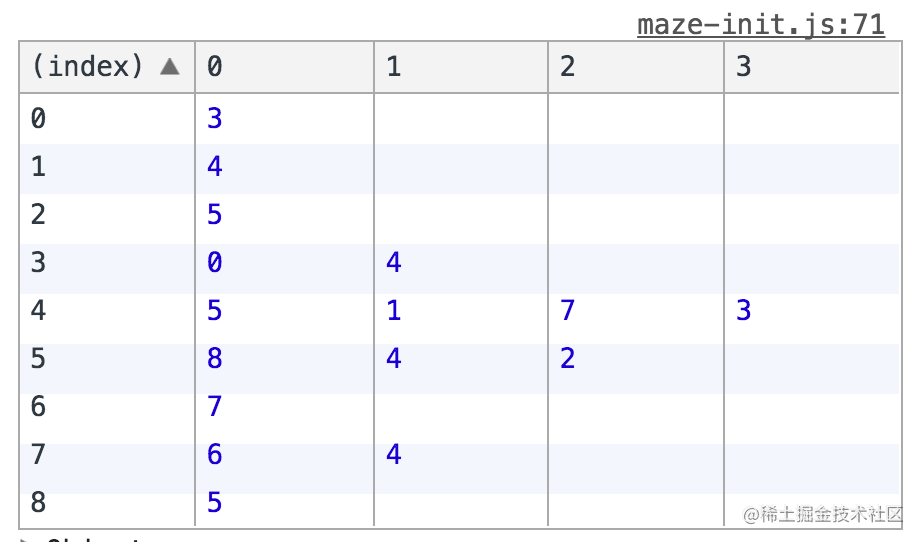``````draw(){
var cellWidth = this.canvas.width / this.columns,
cellHeight = this.canvas.height / this.rows;
var ctx = this.canvas.getContext("2d");
//translate 0.5个像素，避免模糊
ctx.translate(0.5, 0.5);
for(var i = 0; i < this.cells; i++){
var row = i / this.columns >> 0,
column = i % this.columns;
//画右边的竖线
if(column !== this.columns - 1 && (!linkedMap[i] || linkedMap[i].indexOf(i + 1) < 0)){
ctx.moveTo((column + 1) * cellWidth >> 0, row * cellHeight >> 0);
ctx.lineTo((column + 1) * cellWidth >> 0, (row + 1) * cellHeight >> 0);
}
//画下面的横线
if(row !== this.rows - 1 && (!linkedMap[i] || linkedMap[i].indexOf(i + this.columns) < 0)){
ctx.moveTo(column * cellWidth >> 0, (row + 1) * cellHeight >> 0);
ctx.lineTo((column + 1) * cellWidth >> 0, (row + 1) * cellHeight >> 0);
}
}
//最后再一次性stroke，提高性能
ctx.stroke();
//画迷宫的四条边框
this.drawBorder(ctx, cellWidth, cellHeight);
}复制代码``````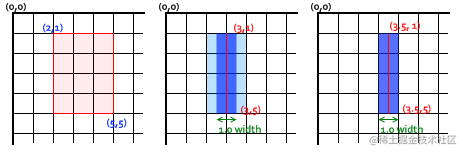``````draw(){
var canvasBuffer = document.createElement("canvas");
canvasBuffer.width = this.canvas.width;
canvasBuffer.height = this.canvas.height;
var ctx = canvasBuffer.getContext("2d");
ctx.translate(0.5, 0.5);
for(var i = 0; i < this.cells; i++){

}
ctx.stroke();
this.drawBorder(ctx, cellWidth, cellHeight);
console.log("draw");
this.canvas.getContext("2d").drawImage(canvasBuffer, 0, 0);
}复制代码``````

``````const column = 50,
row = 50;
var canvas = document.getElementById("maze");
var maze = new Maze(column, row, canvas);

console.time("generate maze");
maze.generate();
console.timeEnd("generate maze");
console.time("draw maze");
maze.draw();
console.timeEnd("draw maze");复制代码``````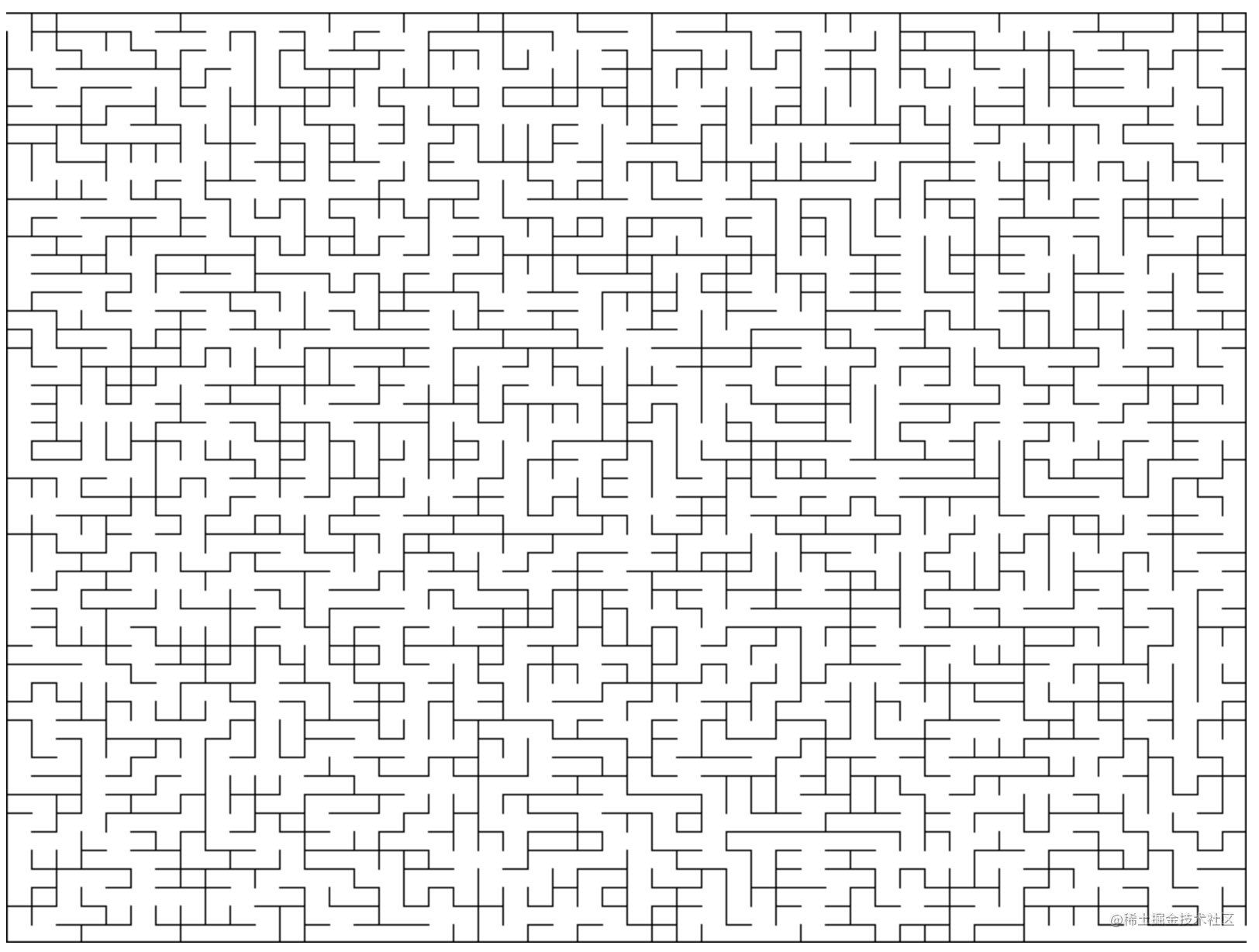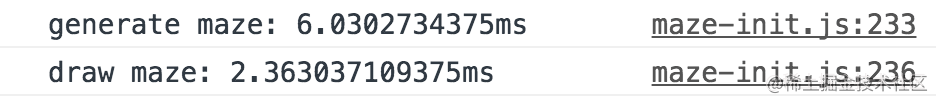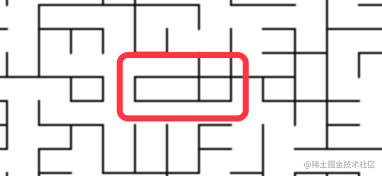``````linkedToFirstCell(){
for(var i = 1; i < this.cells; i++){
if(!this.unionSets.sameSet(0, i))
return false;
}
return true;
}复制代码``````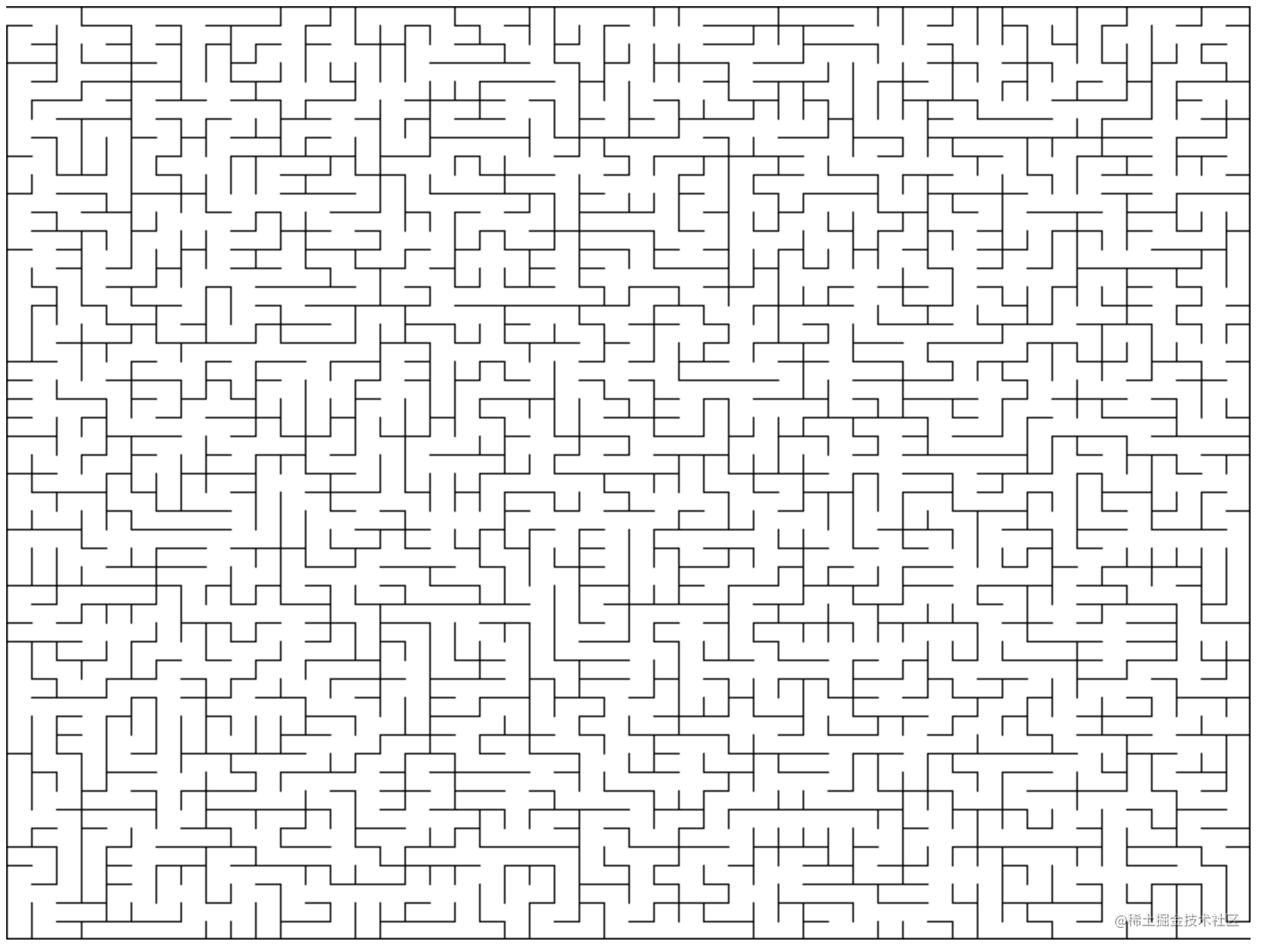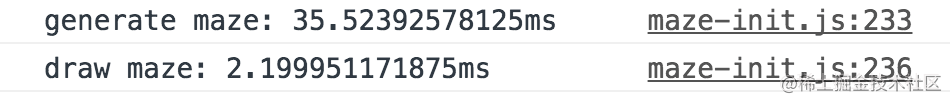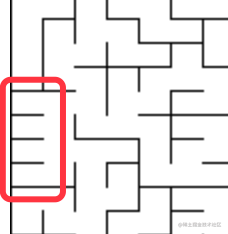## 3. 用最短路径算法找到迷宫的出路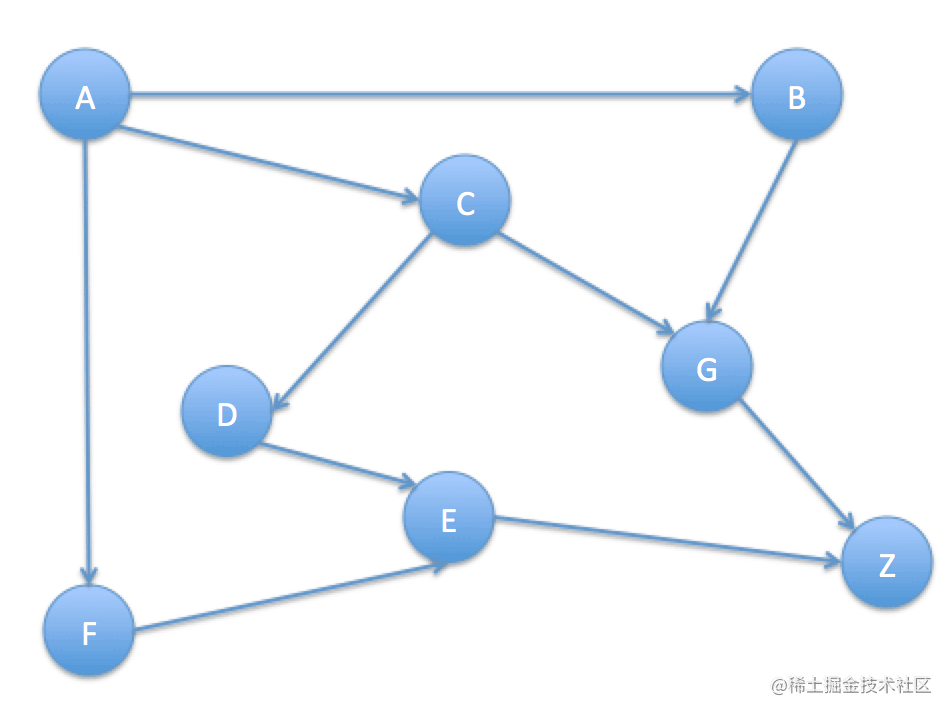``````calPath(){
var pathTable = new Array(this.cells);
for(var i = 0; i < pathTable.length; i++){
pathTable[i] = {known: false, prevCell: -1};
}
pathTable.known = true;
var map = this.linkedMap;
//用一个队列存储当前层的节点，先进队列的结点优先处理
var unSearchCells = ;
var j = 0;
while(!pathTable[pathTable.length - 1].known){
while(unSearchCells.length){
var cell = unSearchCells.pop();
for(var i = 0; i < map[cell].length; i++){
if(pathTable[map[cell][i]].known) continue;
pathTable[map[cell][i]].known = true;
pathTable[map[cell][i]].prevCell = cell;
unSearchCells.unshift(map[cell][i]);
if(pathTable[pathTable.length - 1].known) break;
}
}
}
var cell = this.cells - 1;
var path = [cell];
while(cell !== 0){
var cell = pathTable[cell].prevCell;
path.push(cell);
}
return path;
}复制代码``````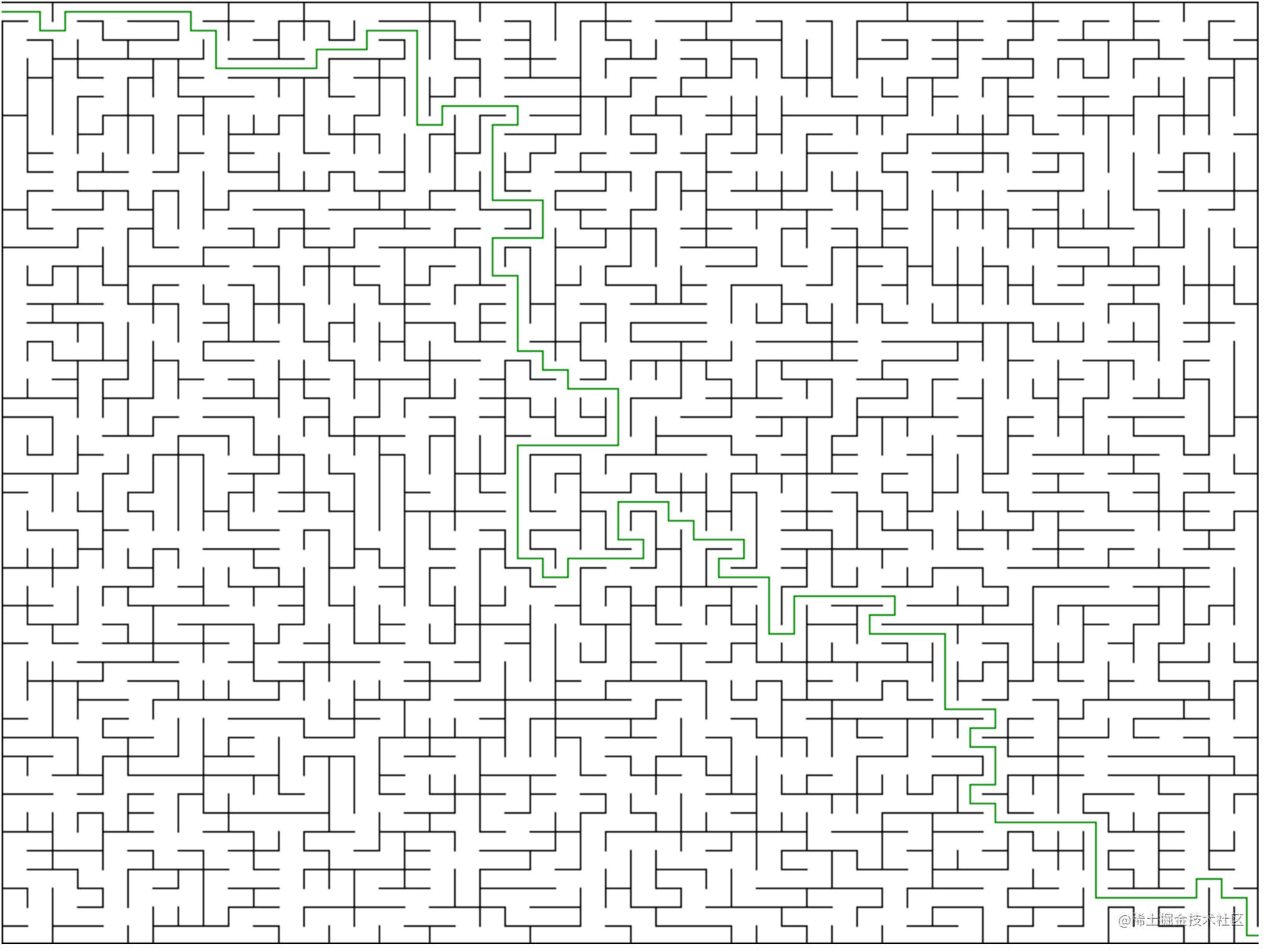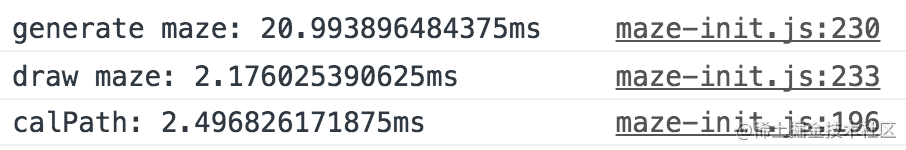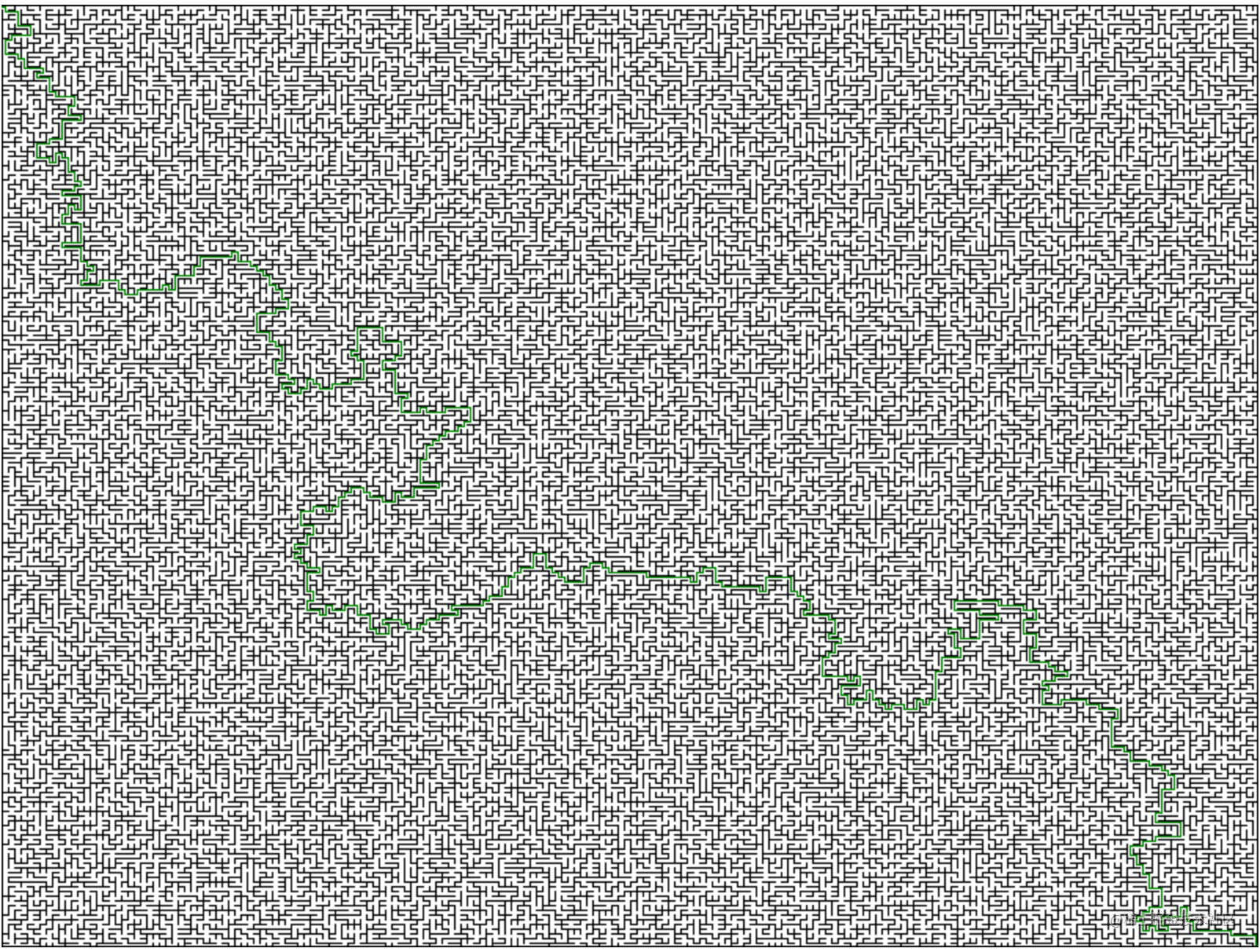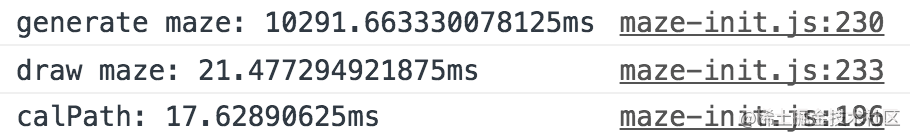## 4. 用WASM生成迷宫

``````struct Data{
int *set;
int columns;
int rows;
int cells;
} data;

void Set_union(int root1, int root2){
int *set = data.set;
if(set[root1] < set[root2]){
set[root2] = root1;
} else {
if(set[root1] == set[root2]){
set[root2]--;
}
set[root1] = root2;
}
}

int Set_findSet(int x){
if(data.set[x] < 0) return x;
else return data.set[x] = Set_findSet(data.set[x]);
}复制代码``````

``````#include <emscripten.h>

EMSCRIPTEN_KEEPALIVE //这个宏表示这个函数要作为导出的函数
int **Maze_generate(int columns, int rows){
Maze_init(columns, rows);
Maze_doGenerate();
//return Maze_getJSONStr();
}复制代码``````

emcc maze.c -Os -s WASM=1 -o maze-wasm.html

``````<script src="maze-wasm.js"></script>
<script src="maze.js"></script>复制代码``````

``````var maze = new Maze(column, row, canvas);
var ptr = Module._Maze_generate(column, row);
maze.draw();
});复制代码``````

``````var linkedMapPtr = Module.ccall("Maze_generate", "number",
["number", "number"], [column, row]);复制代码``````

``````var mazeGenerate = Module.cwrap("Maze_generate", "number",
["number", "number"]);
var linkedMapPtr = mazeGenerate(column, row);复制代码``````

``````function readInt32Array(ptr, length) {
var linkedMap = new Array(length);
for(var i = 0; i < length; i++) {
var subptr = Module.getValue(ptr + (i * 4), 'i32');
var neiborcells = [];
for(var j = 0; j < 4; j++){
var value = Module.getValue(subptr + (j * 4), 'i32');
if(value !== -1){
neiborcells.push(value, 'i32');
}
}
}
}复制代码``````

``````var count = 50;
console.time("JS generate maze");
for(var i = 0; i < count; i++){
var maze = new Maze(column, row, canvas);
maze.generate();
}
console.timeEnd("JS generate maze");

console.time("WASM generate maze");
for(var i = 0; i < count; i++){
var maze = new Maze(column, row, canvas);
var ptr = Module._Maze_generate(column, row);
var linkedMap = readInt32Array(ptr, column * row);
}
console.timeEnd("WASM generate maze");
})复制代码``````